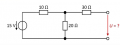Simple circuit calculating the voltage

arhzz1

Joined Oct 21, 2020
42
Hello!

Consider this circuitAs can be seen in the picture I want to calculate the voltage(the red one).I think the english term for this is terminal voltage? Maybe not correct me if I am wrong;

Now this was my approach. First I wanted to calculate the resistance of the entire circuit and I did it like this. The resistor of 10 Ohm (lets call him R1) and the 20 Ohm resistor (lets call him R2) are parallel; So their voltage should be R12 = 6,66 Ohm. Than that should be in series with the 30 Ohm resistor (R3) and the total resitance of the circuit should be Rtotal = 36,6 Ohm

Now I can calculate the current I,as I = Uq(15V)/Rtotal = 0,40 A.

So now I used the Kirchoffs 1 and 2nd law to set up my equations. For the current law (I think that is the english term) I said that the current leaving R1 and flowing into the upper junction is the same as the currents leaving the upper junction; hence

I1 = I2 + I3;

For the Kirchoffs Loop Law I made two loops; for the left one I got this

U1 + U2 - Uq = 0; (if we take the direction clockwise)

For the right one I got this

U3 +U - U2 = 0;

Now since I know the current I should be able to calculate U1 as U1 = R1 * I; that should be 4V. Now I can solve my first loop equation (the left one)

4 + U2 - 15 = 0 --> U2 = 9V

Okay so now I know; U1 = 4V, U2 = 9V I1 = 0,40A

To solve my second loop I need U3. I can calculate U3 as U3 = I3 * R3. I can get I3 if I find I2,for that I used the fact that I know U2 and R2;

I2 = U2/R2 = 0,45A. Now I plug that into the very first equation I1 = I2 + I3 --> I3 = -0,05 A.

Plug that into U3 = -1,5 V

And now plug this into my second loop equation

-1,5 + U - 9 = 0 --> U = 10,5 V

Now the solution should be 10V. Where did I make mistake? I double checked my work by the equations and they should be correct (considering the direction I choose at the loops).Did I make an error at the beginning? Maybe I calculate Rtotal wrong;

Many thanks!

crutschow

Joined Mar 14, 2008
28,538
Hint:
You are making the problem too hard.
Since the 30Ω resistor has no current through it, then it has no effect on the terminal voltage.

arhzz1

Joined Oct 21, 2020
42
Okay so I can just leave it out leaving me with R1 and R2? But that would mean that the terminal voltage is simply the voltage of the source? Since I'd have one loop with only these two voltages,Or would their be a voltage drop at R1 and R2?

boostbuck

Joined Oct 5, 2017
99
R1 and R2 are configured as a voltage divider on the source. If you calculate the current, the voltage should be apparent.

•arhzz1

arhzz1

Joined Oct 21, 2020
42
R1 and R2 are configured as a voltage divider on the source. If you calculate the current, the voltage should be apparent.
Ahhhhh voltage divider, I am having trouble spotting it even tho it is staring me right in the face;

I just used the formula for the voltage divider; U = Uq * R2/R1+R2 and I get the wanted voltage;

Although I am not sure what you meant by "if you calculate the current the voltage should be apparent" can you elaborate on that a bit?

Thanks!

boostbuck

Joined Oct 5, 2017
99
You can cross-check the answer you obtained using the formula of resistance ratios by determining the current through the divider and calculating the voltage drops across R1 and R2.

15V over (10 + 20)R = 0.5A -> 0.5A x 20R = 10V

•arhzz1

arhzz1

Joined Oct 21, 2020
42
You can cross-check the answer you obtained using the formula of resistance ratios by determining the current through the divider and calculating the voltage drops across R1 and R2.

15V over (10 + 20)R = 0.5A -> 0.5A x 20R = 10V

Perfect thanks! Also a question. If I were not to neglect the R3,would I been able to solve this using the voltage divider but consider it to be a loaded voltage divider? where R3 would be that load?

boostbuck

Joined Oct 5, 2017
99
Yes. The 30R (plus any additional load resistance) would be in parallel with the 20R.

Assuming the 30R is a completed circuit, of course. As drawn with one end unconnected, the current through it and voltage drop across it is zero.

Last edited:
•arhzz1

arhzz1

Joined Oct 21, 2020
42
Yes. The 30R (plus any additional load resistance) would be in parallel with the 20R.

Assuming the 30R is a completed circuit, of course. As drawn with one end unconnected, the current through it and voltage drop across it is zero.
Perfekt thanks

Audioguru again

Joined Oct 21, 2019
4,117
R1 and R2 are not in parallel. They are obviously in series!
Then the 15V is across 10 ohms plus 20 ohms causing a current of 0.5A and the voltage across the 20 ohms is 0.5A x 20 ohms= 10V.
The 30 ohms resistor at the output has no load so it has no voltage drop causing the output to ne 10V.